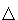# Parabola As Envelope of Straight Lines

Parabola is a conic defined by its focal property: there is a point - focus - and a line - directrix - and parabola is the locus of points equidistant from the focus and the directrix. It is known that the tangent to parabola at a point bisects the angle between the segments joining the point to the focus and the directrix. This leads to another way of obtaining parabola.

### This applet requires Sun's Java VM 2 which your browser may perceive as a popup. Which it is not. If you want to see the applet work, visit Sun's website at https://www.java.com/en/download/index.jsp, download and install Java VM and enjoy the applet.

 What if applet does not run?

The notations here have been borrowed from the discussion on reflective properties of parabola.

The x-axis lies midway between the focus and the directrix. Therefore, the midpoint T of FA' lies on the x-axis. In addition, FT is perpendicular to AT, the tangent at A. In a reversed process, assume a point and a line are given. (We'll take F as a given point and the x-axis as the given line.) Connect the point F to an arbitrary point T on the x-axis and construct a line t perpendicular to FT at T. The family of those straight lines drawn for many points T clearly delineates a parabola. This is the parabola with focus F and the apex on the x-axis. The parabola is said to be the envelope of the family of straight lines {t}. The process of obtaining parabola in this manner is known as the pedal construction [Yates, p. 50]. The x-axis is said to be the pedal of the parabola with respect to its focus.

### Corollary (Parabola by paperfolding)

Now imagine a parabola, its focus and directrix drawn on a sheet of paper. SinceFAA' is isosceles, and AT is its perpendicular bisector, one may fold the paper along AT. The crease thus obtained, which is none other than AT, is a particular case of a mathematical object known as paper line. A paper point is a result of intersection of two paper lines [Martin, Ch. 10]. The whole business of drawing with paper points and lines (there are also paper circles) was introduced in 1983 by T. Sundra Row from India. The first rigorous treatment of paperfolding is attributed to R. C. Yates (1949). The best known axiomatics of paperfolding is due to Humiaki Huzita (1991.)

If, at the outset, the parabola is not given, but only a point and a line, we may produce any number of creases by folding the paper so that the given point falls onto the given line. In time, a parabola will emerge as the envelope of paper lines. Note that it does not matter whether the goal of a particular folding is to place a point on a line, or make the line pass through the point. As a practical matter, if the given line coincides with a paper edge then it is much easier to pursue the latter goal.

### References

1. G. E. Martin, Geometric Constructions, Springer, 1998
2. R. C. Yates, Curves and Their Properties, NCTM, 1974 (J. W. Edwards, 1959)Paper Folding Geometry### Conic Sections > Parabola